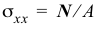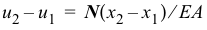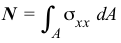# 4.1 Axial Members

In the questions below, select the correct answer.

Name Email
1. Axial strain is uniform across a nonhomogeneous cross section.
2. Axial stress is uniform across a nonhomogeneous cross section.
3. The formulacan be used for finding the stress on a cross section of a tapered axial member.
4. The formulacan be used for finding the deformation of a segment of a tapered axial member.
5. The formulacan be used for finding the stress on a cross section of an axial member subjected to distributed forces.
6. The formulacan be used for finding the deformation of a segment of an axial member subjected to distributed forces.
7. The equationcannot be used for nonlinear materials.
8. The equationcan be used for a nonhomogeneous cross section.
9. External axial forces must be colinear and pass through the centroid of a homogeneous cross section for no bending to occur.
10. Internal axial forces jump by the value of the concentrated external axial force at a section.